# Accessible STEM

### Anticipating and resolving barriers

Emma Cliffe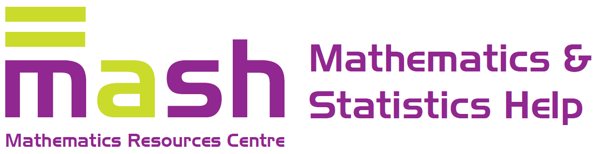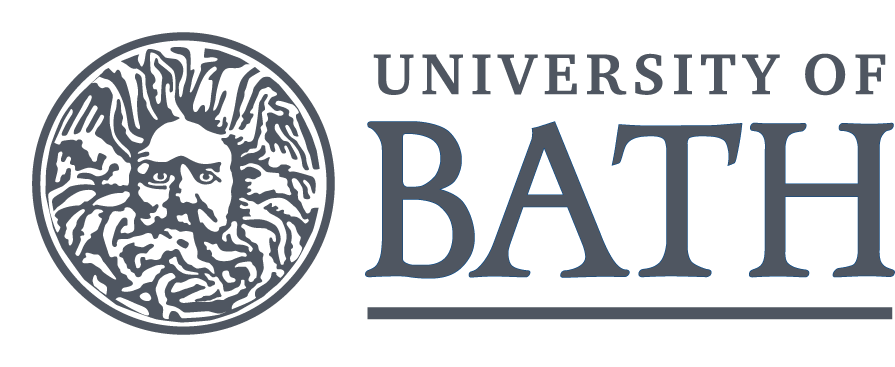# STEM

Science, Technology, Engineering and Mathematics learning may involve substantial quantities of:

• Maths
• Diagrams
• STEM Computing

What are the barriers in this context?

How do we resolve them?

# Conversations

with students and staff

## Maths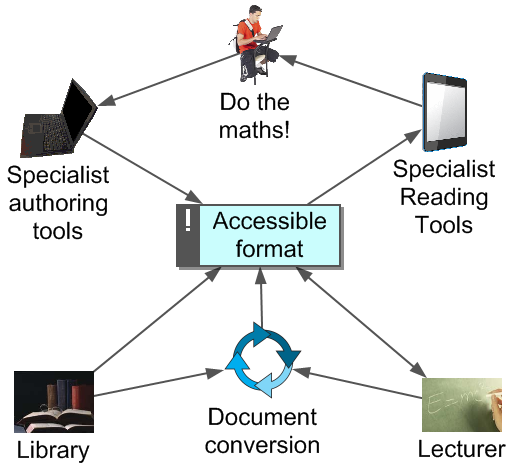### ✓ Got the notes/book

But PDF is a lossy format...

So are most handwriting, print, images, PowerPoint, Ebooks...

Aim for a format which encodes structure:

### Doing and authoring

Using a computer to do/author maths is a skill.

Do staff and students have...

• appropriate software?
• effective working methods?
• methods to independently and efficiently produce output others can read?

Does Disabled Students' Allowance (DSA) provision meet these needs?

### Software

Rapid change/technical barriers... DSA provision?

## Diagrams

An example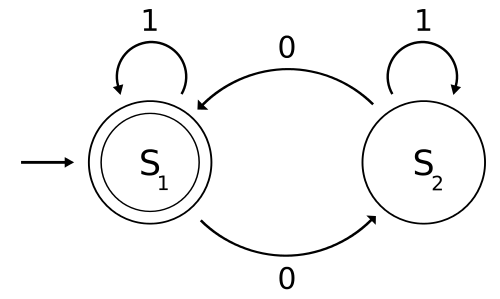Two circles labelled $$S_1$$ and $$S_2$$. Circle $$S_1$$, on the left, is drawn with a double line and has an arrow pointing at it, horizontally from the left. Circle $$S_2$$, on the right is drawn with a single line. An arc labelled $$1$$ goes from $$S_1$$ to itself. An arc labelled $$0$$ goes from $$S_1$$ to $$S_2$$. An arc labelled $$1$$ goes from $$S_2$$ to itself. An arc labelled $$0$$ goes from $$S_2$$ to $$S_1$$.FSM with states $$\{S_1, S_2\}$$ where $$S_1$$ is initial and final. Transitions: \begin{align*} S_1 &\rightarrow_1 S_1,\ S_1 \rightarrow_0 S_2\\ S_2 &\rightarrow_1 S_2,\ S_2 \rightarrow_0 S_1 \end{align*}Formal definition:

\begin{align*} Q &= \{S_1, S_2\}\\ \Sigma &= \{0, 1\}\\ q_0 &= S_1\\ F &= \{S_1\} \end{align*}

$\delta = \begin{array}{c|c|c} & 0 & 1 \\ \hline S_1 & S_2 & S_1\\ \hline S_2 & S_1 & S_2 \end{array}$Machine equivalent to regular expression: $(1 + 0(1^*)0)^*$

Machine which accepts inputs containing an even number of $$0$$s and any number of $$1$$s.FSM with states $$\{S_1, S_2\}$$ where $$S_1$$ is initial and final. Transitions: \begin{align*} S_1 &\rightarrow_1 S_1,\ S_1 \rightarrow_0 S_2\\ S_2 &\rightarrow_1 S_2,\ S_2 \rightarrow_0 S_1 \end{align*}

Formal definition: \begin{align*} Q &= \{S_1, S_2\}\\ \Sigma &= \{0, 1\}\\ q_0 &= S_1\\ F &= \{S_1\}\\ \delta &= \begin{array}{c|c|c} & 0 & 1 \\ \hline S_1 & S_2 & S_1\\ \hline S_2 & S_1 & S_2 \end{array} \end{align*}

Machine equivalent to regular expression: $(1 + 0(1^*)0)^*$

Machine which accepts inputs containing an even number of $$0$$s and any number of $$1$$s.

Two circles labelled $$S_1$$ and $$S_2$$. Circle $$S_1$$, on the left, is drawn with a double line and has an arrow pointing at it, horizontally from the left. Circle $$S_2$$, on the right is drawn with a single line. An arc labelled $$1$$ goes from $$S_1$$ to itself. An arc labelled $$0$$ goes from $$S_1$$ to $$S_2$$. An arc labelled $$1$$ goes from $$S_2$$ to itself. An arc labelled $$0$$ goes from $$S_2$$ to $$S_1$$.digraph finite_state_machine {
rankdir=LR;
size="8,5"
node [shape = doublecircle]; S_1
node [shape = circle];
S_1 -> S_2 [ label = "0" ];
S_1 -> S_1 [ label = "1" ];
S_2 -> S_1 [ label = "0" ];
S_2 -> S_2 [ label = "1" ];
}



### Consuming and creating

Can staff and students...

• flexibility communicate diagrammatic content in context?
• effectively encode and use structural relationships?
• use subject specific methods of production?

What specialist knowledge and skills are required?

What are the costs?

Does Disabled Students' Allowance (DSA) provision meet these needs?

### Software and hardware

Requirement to specifically consider diagrams often overlooked by DSA process...

• Context appropriate descriptions by subject specialists
• Low tech drawing aids e.g. Plastic embossing film
• Specialist production methods e.g. tactile diagrams, audio-tactile systems, 3D printing
• Vector graphics editors e.g. to output SVG
• Specialist subject software (is it accessible?)
• Encodings e.g. LaTeX, Graphviz
• Tablet computer, digital pens?

## STEM Computing

Emma! Why isn't it working?!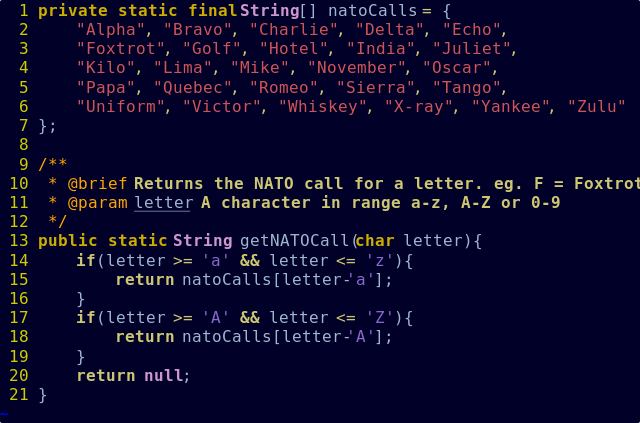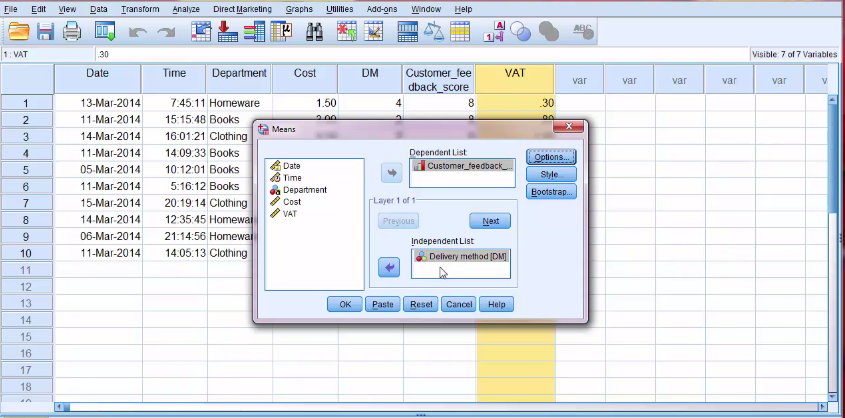### Programming can be powerful

or a source of substantial difficulty, frustration and stress

• Holding flow in memory
• Remembering vocab, patterns etc.
• Avoiding and correcting typos
• Commenting including spelling, grammar etc.
• Version control
• Structured approach to testing and debugging
• Managing interaction between elements

### But we knew that already!

Introduce a selection of appropriate industry standard, high-quality software and tools.

After all, we use these tools for a reason.

Facilitate students to find an approach and tools which work for them.

Does Disabled Students' Allowance (DSA) provision meet these needs?

### Flexible approach to toolset

Introduce, provide, support and encourage use of a selection of

• Text editors
• Integrated Development Environments
• Interfaces e.g. command line, graphical
• Version control and build management systems
• Testing methods
• Project management techniques

Are staff and students ready and able to get involved in tool exploration?

### User interfaces

Emma! It isn't accessible!

It is often assumed that STEM specific software user interfaces are accessible.

The lecturer, department and institution needs to proactively explore this question.

• Is accessibility considered during software procurement?
• What about free software selection?
• Is independent advice and guidance sought?
• Are problems reported and followed up with publishers?

Are staff aware of alternatives and can adjust teaching? E.g. use of command line only interfaces to R, MatLab, Maple

Does institution infrastructure and DSA support use of assistive technology and STEM user interfaces in combination?

# Anticipating and resolving barriers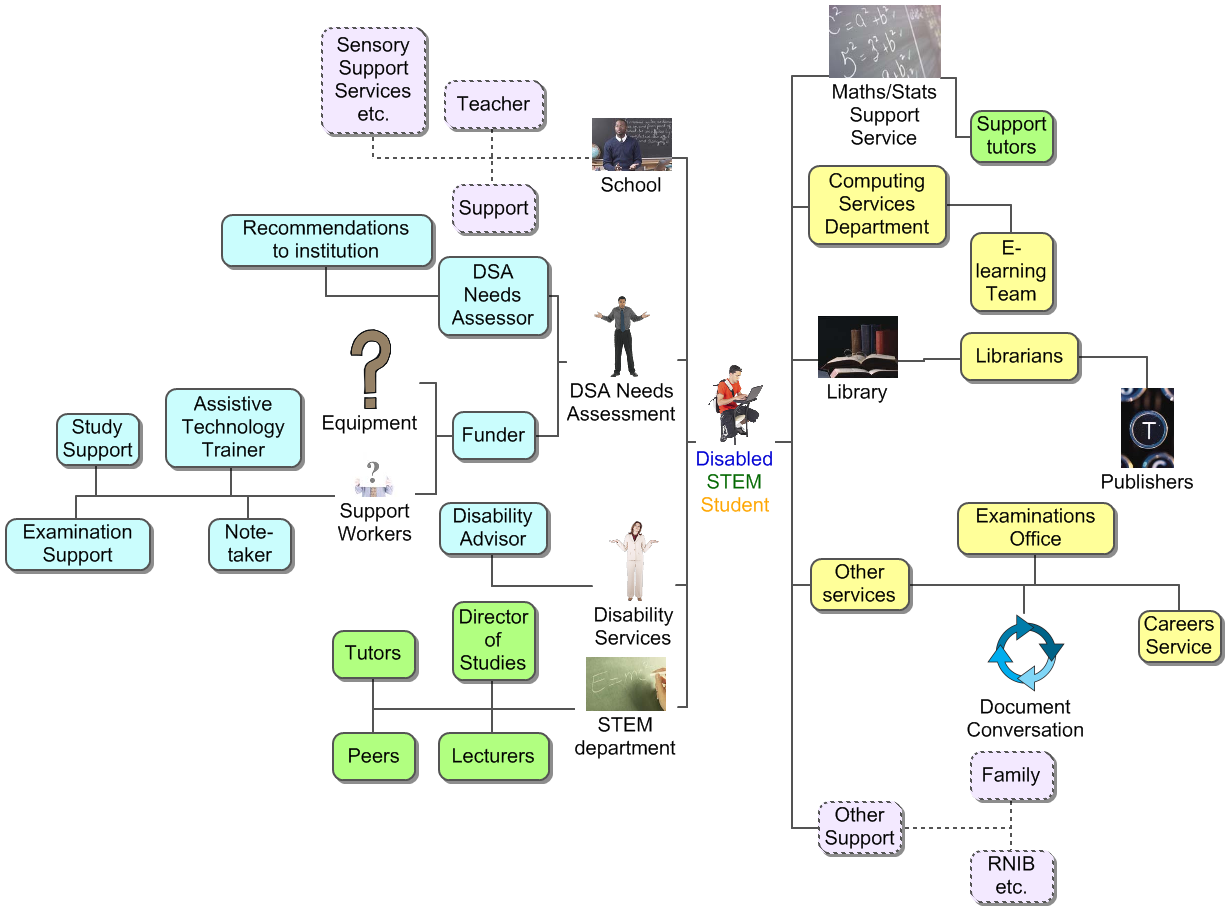## Who...

• anticipates these issues?
• plans for and resolves these barriers?
• might make faulty assumptions?

Where does the expertise lie?

"If you have been reliant on support at school, transition to the university might be a challenge."
"Lack of anticipation is a barrier, you need to prepare in advance."
"You have to be 'up and running' before the course starts."

### School/College

"The difficulties of the subject make it difficult for the teacher to prepare the student. You can't expect every school to have expertise."
"There needs to be collaboration between schools and universities."

### University

"Students need to be able to identify their needs and be clear about it."
"Current recommendations do not fully reflect student's needs."
"If these developments [access methods and technologies] are subject specific, how can disability specialists access this information?"

## How?

### Collaboration and infrastructure

• Student understands what has worked for them before.
• School and college helps prepare for transition.
• Professional services provide infrastructure and support and enable students.
• STEM specialists bring understanding of their subject, how it is learnt, practised and communicated.
"Not many people in the country know how to use the systems"Can we change that?

# Discussion

Thanks for listening. Questions and discussion welcome.

These slides are available at:
http://people.bath.ac.uk/cspehj/slides/BCS/